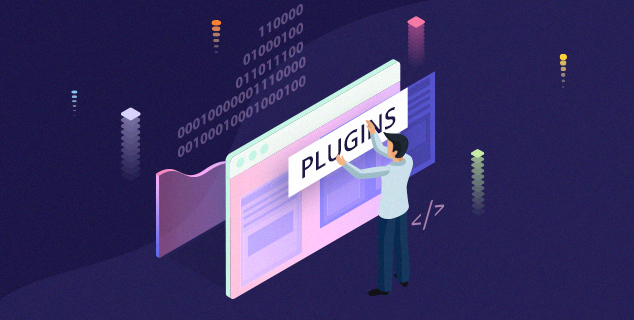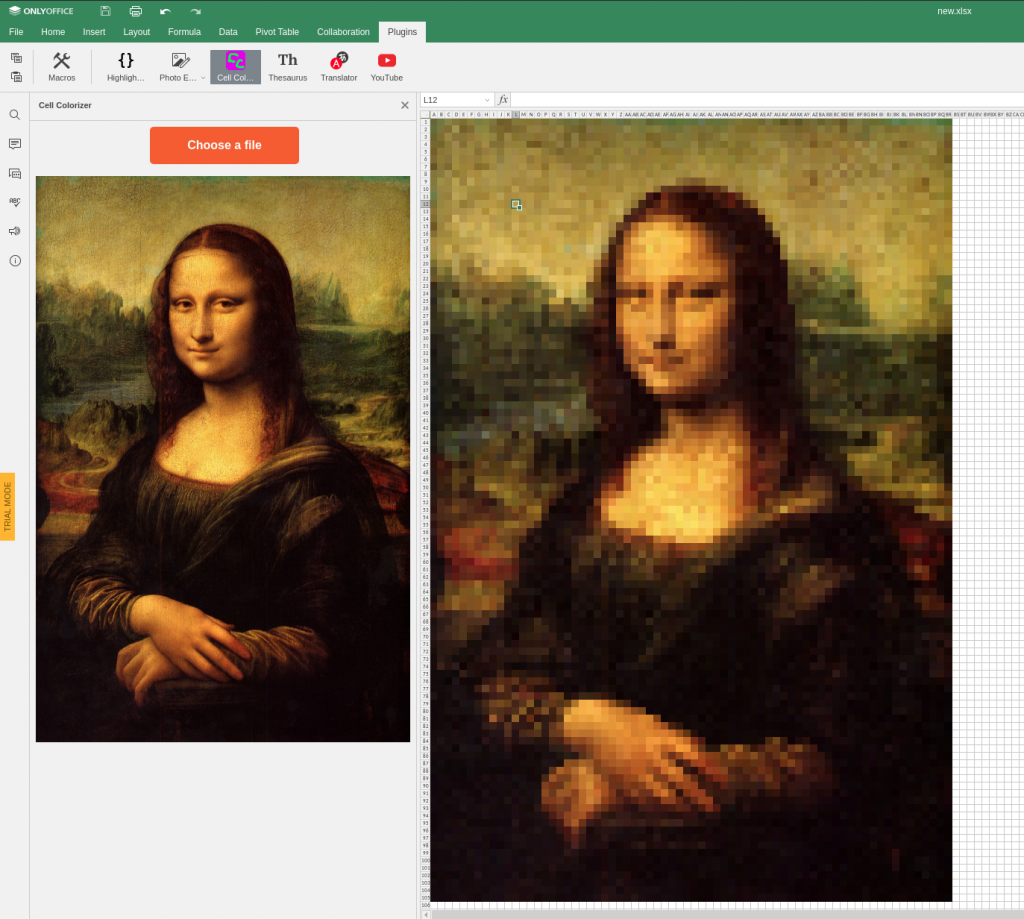# Plugin to fill cells with color and turn your worksheet into an image

8 June 2020

Hello!

The cell colorizer plugin fills cells with color to display images in spreadsheets. One of our engineers created it just for fun and explained the development process. We hope that this example will inspire you to create your own plugins.How it works

1. You upload an image (jpg, png, bmp).
2. The plugin fills cells in a spreadsheet with its pixels color.
3. You get the image in your spreadsheets (it looks weird but somewhat enjoyable).

You can find the plugin code in this repo.

Plugin structure

This plugin (as well as any plugin for ONLYOFFICE editors) contains these parts:

1. HTML file with plugin interface.
2. js file with plugin code.
3. JSON file with plugin config.

Take these template files if you want to create your own plugin.

Changing config

Let’s work with config.json for a start.

It’s convenient to have a plugin interface on the left panel. To do so, let’s add these parameters:

``````"isVisual" : true,
"isInsideMode" : true
``````

“isVisual” means that the plugin will have its own interface, “isInsideMode” means that it will appear on the left panel, not in a modal window.

The cell colorizer plugin works for spreadsheets only, so let’s remove all the values from “EditorsSupport” parameter, except for “cell”. This way the plugin will appear only in the spreadsheet editor.

Changing HTML

`````` <input type="file" id="load-input" />
<img id="input-image">
``````

In order not to fill too many cells (we want to colorize the visible area only), we need to scale the image. This is a task for canvas that will also help us get the right colors for pixels.

``[object Object]``
Creating code

``````const canvas = get_canvas();
const image = get_image();
function get_image() {
return document.getElementById('input-image');
}
function get_canvas() {
return document.getElementById('canvas');
}``````

After a user uploads image, we need to display it in our interface and then start drawing on canvas and filling cells.

Let’s create an event for displaying image:

``````
image.src = URL.createObjectURL(event.target.files);
drawImage();
}
};
``````

After the image has been displayed in the plugin, we draw it on canvas.

The image can be really big, thousands of pixels in width. We don’t want to fill that many cells. Let’s downsize the canvas.

For example, if we want to fill only 70 cells for the image width and any number of cells for its height, we need to set canvas width to 70. Canvas height should have the value `image_height * 70 / image.width`.

After that, you can paint over the entire canvas with the image and take the array of canvas pixels to fill the cells.

``````
function drawImage() {
canvas.width = 70;
let scale = canvas.width/ image.width
canvas.height = image.height * scale;
var ctx = canvas.getContext('2d');
ctx.drawImage(image, 0, 0, canvas.width, canvas.height);
Asc.scope.st = { "data": ctx.getImageData(0, 0, canvas.width,
canvas.height).data.toString(), "width": canvas.width, "height": canvas.height };
filling_cells()
}``````

`Asc.scope.st` – is an object that allows passing data as a string to the `window.Asc.plugin.callCommand` method. Details can be found here.

ctx.getImageData(0, 0, canvas.width, canvas.height).data – here we get an array of pixel colors. Data format is [r1,g1,b1,a1,r2,g2,bg2,a2…].

Let’s add the method to fill the cell with the colors we got from canvas:

``````
function filling_cells() {
window.Asc.plugin.callCommand(function () {
var oWorksheet = Api.GetActiveSheet();
const height = Asc.scope.st['height']
const width = Asc.scope.st['width']
const data = Asc.scope.st['data'].split(',')
let dataIndex = 0
let dataR;
let dataG;
let dataB;
let range;
for (let x = 0; x < Asc.scope.st['height']; x++) {
for (let y = 0; y < Asc.scope.st['width']; y++) {
const alpha = ((data[dataIndex + 3])) / 255
dataR = alpha * data[dataIndex]
dataG = alpha * data[dataIndex + 1]
dataB = alpha * data[dataIndex + 2]
range = oWorksheet.GetRangeByNumber(x, y);
range.SetFillColor(Api.CreateColorFromRGB(dataR, dataG, dataB));
dataIndex = dataIndex + 4
}
// oWorksheet.SetColumnWidth(x, 2);
}
});
}``````

`window.Asc.plugin.callCommand` is the method to work with editors via API. Details here.

Let’s add variables and constants that we are going to work with:

``````  var oWorksheet = Api.GetActiveSheet();
const height = Asc.scope.st['height']
const width = Asc.scope.st['width']
const data = Asc.scope.st['data'].split(',')
let dataIndex = 0
let dataR;
let dataG;
let dataB;``````

After that, we add two nested loops to move between spreadsheet rows and columns:

``````  for (let x = 0; x < Asc.scope.st['height']; x++) {
for (let y = 0; y < Asc.scope.st['width']; y++) {``````

It makes sense a simplified conversion formula from rgba to rgb since the color of the cells is always white:

`````` const alpha = ((data[dataIndex + 3])) / 255
dataR = alpha * data[dataIndex]
dataG = alpha * data[dataIndex + 1]
dataB = alpha * data[dataIndex + 2]``````

After we fill the current cell with color, we move to the next one:

``````
oWorksheet.GetRangeByNumber(x,
y).SetFillColor(Api.CreateColorFromRGB(dataR, dataG, dataB));
dataIndex = dataIndex + 4
``````

After we add the plugin to the editors, it will look like this:Weird, right? Let’s fix the style.

We need to hide the canvas and display the image instead. Also, let’s add change input for the file:

``````
,[object Object]``````

If you want, you can add cell width changing to the cycle, so that the cells become square.

``oWorksheet.SetColumnWidth(x, 2);``

This way the image won’t be too stretched:That’s all!